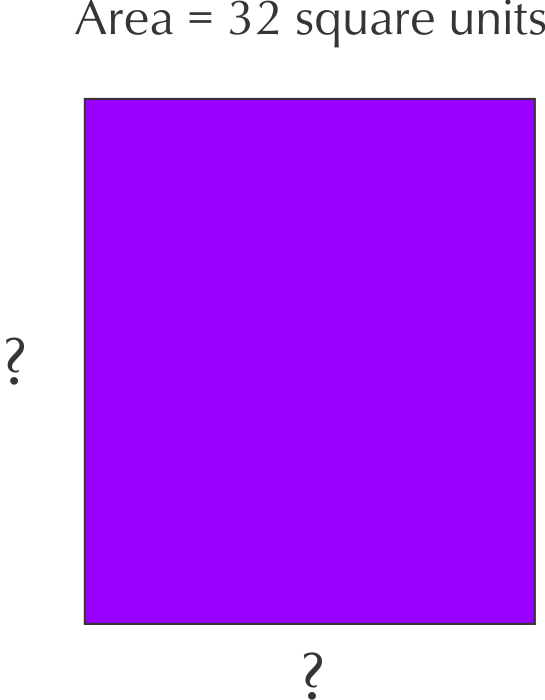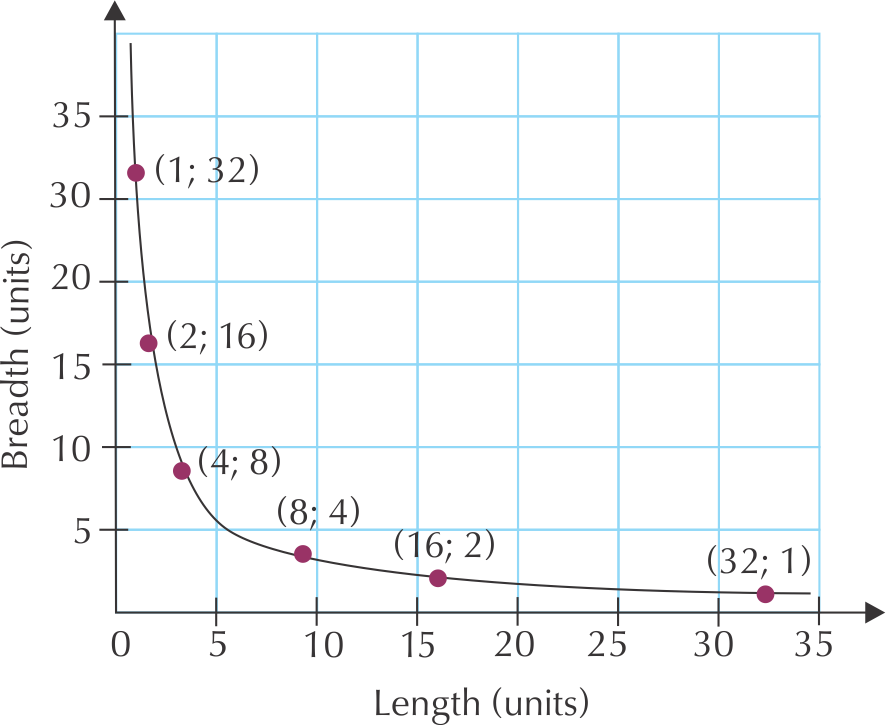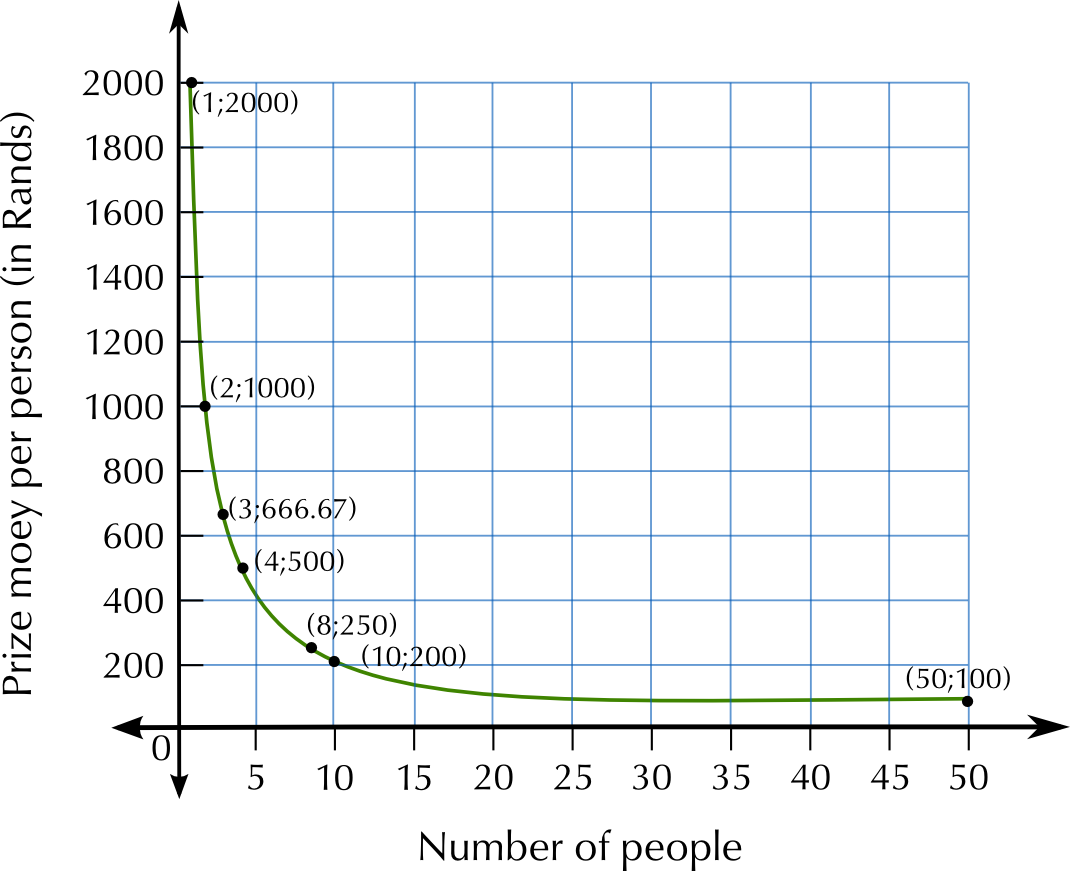Home Practice
For learners and parents For teachers and schools
Textbooks
Full catalogue
Pricing SupportLog in

We think you are located in United States. Is this correct?

# 2.4 Inverse proportion patterns, relationships and graphs

## 2.4 Inverse proportion patterns, relationships and graphs (EMG3G)

### Inverse proportion relationships and graphs (EMG3H)

Some relationships between quantities give patterns that form inverse proportion graphs. How do we recognise an inverse proportion relationship?

Remember from Chapter 1: in an inverse proportion, as one quantity decreases, the other increases OR as one quantity increases, the other decreases.

## Worked example 5: A graph of an inverse proportion

A rectangle has a fixed area of $$\text{32}$$ square units, but the length $$l$$ and breadth $$b$$ can both change. If the length is smaller, the breadth gets bigger, because the area stays the same.1. Complete the following table of possible values for the length and breadth of the rectangle.
 length $$l$$ $$\text{1}$$ $$\text{2}$$ $$\text{4}$$ $$\text{8}$$ $$\text{16}$$ $$\text{32}$$ breadth $$b$$ $$\text{32}$$
2. Draw a graph to show all the possible values of the length and breadth.
3. Is the graph continuous or discrete? Explain.
4. Why does the curve not touch the axes?
1.  length $$l$$ $$\text{1}$$ $$\text{2}$$ $$\text{4}$$ $$\text{8}$$ $$\text{16}$$ $$\text{32}$$ breadth $$b$$ $$\text{32}$$ $$\text{16}$$ $$\text{8}$$ $$\text{4}$$ $$\text{2}$$ $$\text{1}$$
2.3. The graph is continuous, because measurement values are continuous. The curve is solid and not dotted because there are an infinite number of values between the points.
4. The graph doesn't touch the axes because the length and breadth can never be $$\text{0}$$.

The important things to note about the graph of an inverse proportion is that it is a smooth curve, and that the curve never touches the axes. In most cases, we will deal with a positive inverse proportion, because most real life values are positive. But it is possible for them to be negative too.

## Inverse proportion patterns

Exercise 2.3

Lerato decides to get a group of friends together to play a lucky draw game. The bigger the group, the more tickets they can buy, but if they win, they will have to share the prize among more people. The total amount of money is $$\text{R}\,\text{2 000}$$.

If they win the prize money, how much will they have to share?
$$\text{R}\,\text{2 000}$$
How will the number in the group affect the amount each person receives?
The larger the number of people in the group, the smaller the shared amount that each person will receive.
What kind of relationship is this?
An inverse proportional relationship.
Copy and complete the table below including the first column which is the headings for the independent and dependent variables.
 Number of people $$\text{1}$$ $$\text{2}$$ $$\text{3}$$ $$\text{4}$$ $$\text{8}$$ $$\text{10}$$ $$\text{50}$$ Share of the prize money $$\text{2 000}$$ $$\text{1 000}$$
 Number of people $$\text{1}$$ $$\text{2}$$ $$\text{3}$$ $$\text{4}$$ $$\text{8}$$ $$\text{10}$$ $$\text{50}$$ Share of the prize money $$\text{2 000}$$ $$\text{1 000}$$ $$\text{666,67}$$ $$\text{500}$$ $$\text{250}$$ $$\text{200}$$ $$\text{100}$$
Plot a graph of these points to show the relationship.Meryl wants to make a garden bed with an area of $$\text{16}$$ $$\text{m^{2}}$$.Draw up a table to show a few possible length and breadth measurements of the garden bed.

 Breadth (m) $$\text{1}$$ $$\text{2}$$ $$\text{4}$$ $$\text{8}$$ $$\text{16}$$ Length (m) $$\text{16}$$ $$\text{8}$$ $$\text{4}$$ $$\text{2}$$ $$\text{1}$$

Do the measurements have to be whole numbers? Explain.

No. Any two numbers whose product is $$\text{16}$$ can be used, because length and breadth are continuous variables. Whole numbers will be easier to calculate and plot on a graph, however.

Draw a graph to show the relationship between the length and the breadth of the garden bed.